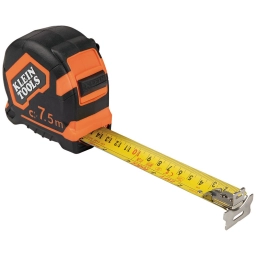# One-fifth 81290

One-fifth of the bridge pillar is embedded in the ground. One-third of its length is in the water, and 1.4 m protrudes above the surface. Determine the length of the bridge pillar.

x =  3 m

### Step-by-step explanation:Did you find an error or inaccuracy? Feel free to write us. Thank you!

Tips for related online calculators
Need help calculating sum, simplifying, or multiplying fractions? Try our fraction calculator.
Do you have a linear equation or system of equations and looking for its solution? Or do you have a quadratic equation?
Do you want to convert length units?